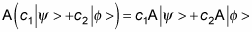##### Quantum Physics For Dummies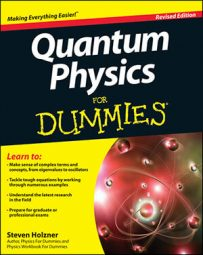In quantum physics, you can use operators to extend the capabilities of bras and kets. Although they have intimidating-sounding names like Hamiltonian, unity, gradient, linear momentum, and Laplacian, these operators are actually your friends.

Taking the product of a bra and a ket,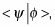is fine as far as it goes, but operators take you to the next step, where you can extract physical quantities that you can measure.

Here’s the general definition of an operator A in quantum physics: An operator is a mathematical rule that, when operating on a ket,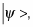transforms that ket into a new ket,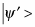in the same space (which could just be the old ket multiplied by a scalar). So when you have an operator A, it transforms kets like this: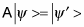For that matter, the same operator can also transform bras: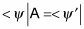Here are some examples of how you can use operators:

• Hamiltonian. Applying the Hamiltonian operator, H (which looks different for every different physical situation) gives you E, the energy of the particle represented by the ket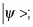E is a scalar quantity: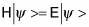• Unity or identity. The unity or identity operator, I, leaves kets unchanged: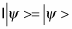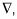works as follows: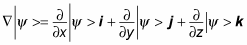• Linear momentum. The linear momentum operator, P, looks like this in quantum mechanics: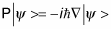• Laplacian. You use the Laplacian operator,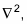which is much like a second-order gradient, to create the energy-finding Hamiltonian operator: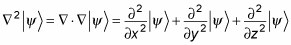Keep in mind that multiplying operators together is not usually the same independent of order, so for the operators A and B,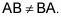And an operator A is said to be linear if